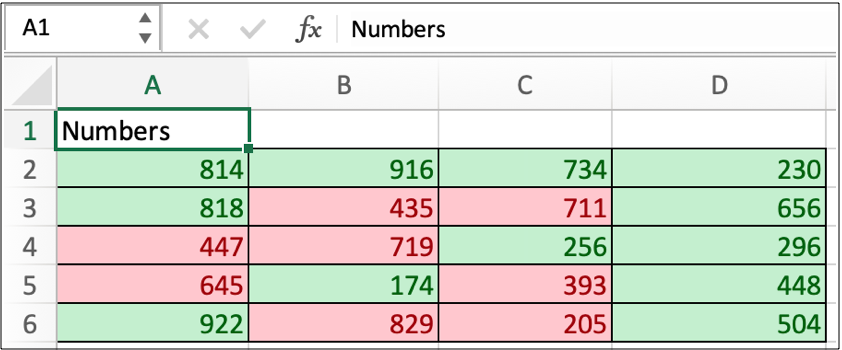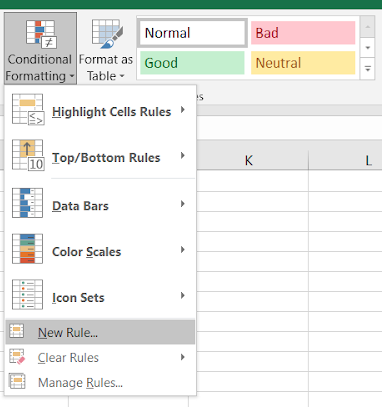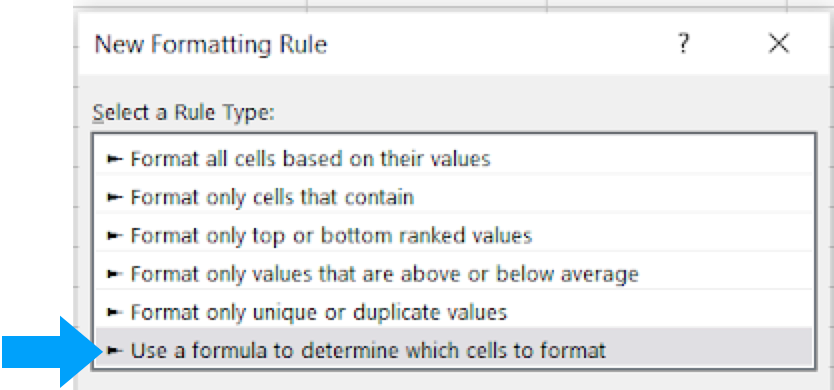# How to format odd number and even number rows in ExcelHow to format odd number and even number rows in Excel

In this article, we will learn How to format odd numbers and even number rows in Excel.

Scenario :

In data analysis and MIS reporting, we often get the need to highlight the odd and even number rows. This helps us to easily identify focus areas. For example applying a new format to the rows where cell value is either odd. Or another thing could be applying format to even numbered or odd numbered rows. You don't worry about

In this article, we will learn how we can highlight the even or odd numbers in Excel data sets, using conditional formatting.

Conditional formatting formula in Excel

Conditional formatting allows you to apply the formatting basis on the cell values such as colours, icons and data bars. To access Conditional formatting in excel follow the below steps.

Select the data. Go to Home > Conditional Formatting > New rule > Use a formula to determine which cells to format.

This will open up a dialog box where you can use the

Divisible by 2 in excel

Divisibility by 2 means the number is an even number. In mathematical terms when a number is divided by 2 and it returns 0. So the number is divisible by 2 and is an even number. In Excel use the ISEVEN function to find the even numbers in the list.

Formula :

 =ISEVEN(cell_ref)

The above function returns TRUE or FALSE

Not Divisible by 2 in excel

Not Divisible by 2 means the number is an Odd number. In mathematical terms when a number is divided by 2 and returns 1 . So the number is not divisible by 2 and is an odd number. In Excel use the ISODD function to find the even numbers in the list.

Formula :

 =ISODD(cell_ref)

The above function returns TRUE or FALSE

Divisible by any other number

You can use this method to check divisibility of any number by any number. MOD function in Excel returns the integer remainder value. So the below formula checks if the MOD function returns 0 (divisible), the formula returns True or if the MOD function returns any other value than 0 then the formula returns FALSE.

Formula :

 =MOD(Dividend,divisor)=0

MOD function returns remainder value but the formula checks if its 0 or not.

Example :

All of these might be confusing to understand. Let's understand how to use the function using an example. Here we have some numbers and we need to check the odd numbers in excel. So first we select the cells which we need to format

Go to home > conditional formatting > New ruleThis will open a New formatting rule dialog box. Select the Use a formula to determine which cells to format.Use the formula:

 =ISODD(A2) #first cell reference with no dollar characters

Click OkAs you can see in the above image all the odd values are formatted with light red color. Now we like to see the even numbers formatted as in green colors.

Check even numbers using ISEVEN function

we need to check the even numbers in excel. So first we select the cells which we need to format

Go to home > conditional formatting > New ruleThis will open a New formatting rule dialog box. Select the Use a formula to determine which cells to format.Use the formula:

 =ISEVEN(A2) #first cell reference with no dollar characters

Click OkAs you can see in the above image all the Even values are formatted with light green color. Whereas odd numbers are formatted as red. Now your eyes can visualize the distribution.

Here are all the observational notes using the formula in Excel
Notes :

1. The formula only works with numbers only.
2. The two ranges provided in the formula must have the same number of rows or the formula returns error.
3. MOD function takes two input values and returns single output (remainder)
4. Operators like equals to ( = ), less than equal to ( <= ), greater than ( > ) or not equals to ( <> ) can be performed within a formula applied, with numbers only.

Hope this article about How to format odd number and even number rows in Excel is explanatory. Find more articles on calculating values and related Excel formulas here. If you liked our blogs, share it with your friends on Facebook. And also you can follow us on Twitter and Facebook. We would love to hear from you, do let us know how we can improve, complement or innovate our work and make it better for you. Write to us at info@exceltip.com.

Related Articles :

Find the partial match number from data in Excel : find the substring matching cell values using the formula in Excel.

How to Highlight cells that contain specific text in Excel : Highlight cells based on the formula to find the specific text value within the cell in Excel.

Conditional formatting based on another cell value in Excel : format cells in Excel based on the condition of another cell using some criteria in Excel.

IF function and Conditional formatting in Excel : How to use IF condition in conditional formatting with formula in Excel.

Perform Conditional Formatting with formula 2016 : Learn all default features of Conditional formatting in Excel.

Conditional Formatting using VBA in Microsoft Excel : Highlight cells in the VBA based on the code in Excel.

Popular Articles :

50 Excel Shortcuts to Increase Your Productivity : Get faster at your tasks in Excel. These shortcuts will help you increase your work efficiency in Excel.

How to use the VLOOKUP Function in Excel : This is one of the most used and popular functions of excel that is used to lookup value from different ranges and sheets.

How to use the IF Function in Excel : The IF statement in Excel checks the condition and returns a specific value if the condition is TRUE or returns another specific value if FALSE.

How to use the SUMIF Function in Excel : This is another dashboard essential function. This helps you sum up values on specific conditions.

How to use the COUNTIF Function in Excel : Count values with conditions using this amazing function. You don't need to filter your data to count specific values. Countif function is essential to prepare your dashboard.

Terms and Conditions of use

The applications/code on this site are distributed as is and without warranties or liability. In no event shall the owner of the copyrights, or the authors of the applications/code be liable for any loss of profit, any problems or any damage resulting from the use or evaluation of the applications/code.